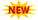WBSSC Krishi Prayukti Sahayak Recruitment || TSSPDCL AE Notification || PPSC Naib Tehsildar Admit Card ||

# NCERT Solutions For Class 11 Statistics For Economics

NCERT Solutions For Class 11 Statistics For Economics pdf is available here. If you are looking for the NCERT Solutions of Class 11 Statistics For Economics then you find answers here. Our experts came here to help students in finding those solutions and explained them in detailed step-by-step explanations. The answers to each chapter in Class 11 Economic Statistics have provided here in the given below links. You can easily browse through different chapters and select the needy one. You can also read this NCERT Class 11 Statistics For Economics Solutions through Online mode. You should practice well for the examination to score good marks. These NCERT Solutions are easy to understand and can solve in exams without any fear. This will help in other exams like JEE, Mains, Advance, UPSC, BIITSAT, and many Competitive exams.

## Statistics For Economics- NCERT Solutions For Class 11 Statistics

NCERT Solutions For Class 11 statistics has provided a  large number of problems to be solved. And it considers 8 chapters. You can download the Chapter-wise Solutions pdf by following the below links.

• Chapter 1 Introduction
• Chapter 2 Collection of Data
• Chapter 3 Organisation of Data
• Chapter 4 Presentation of Data
• Chapter 5 Measures of Central Tendency
• Chapter 6 Measures of Dispersion
• Chapter 7 Correlation
• Chapter 8 Index Numbers

### NCERT Solutions Class 11 Statistics For Economics Chapter 1 Introduction

Chapter 1 shows an introduction to the  NCERT Solutions Class 11 Economics Chapter 1 has covered the topics like What is economics? Meaning, Scope, functions, and importance of statistics in Economics

### NCERT Solutions Class 11 Statistics For Economics Chapter 2 Collection of Data

This chapter tells us about the struggles of a stagnant economy and underdevelopment that affected the Indian Economy before independence and is examined and explained in detail. Class 11 Economics NCERT Solutions has main concepts such as Sources of data, methods of collecting data, some important sources of secondary data, Census of India, and national sample Survey Organisation.

### NCERT Solutions Class 11 Statistics For Economics Chapter 3 Organisation of Data

In this chapter 3, you will learn how to organize the data and how to retrieve data in easy ways. NCERT Solutions For Class 11 statistics Chapter 3 has explained the Meaning, types of variables, and frequency distribution.

### NCERT Solutions For Class 11 Economics Statistics Chapter 4 Presentation of Data

In this chapter, you will learn about the Presentation of Data. The main concepts described in Class 11 Economics for Statistics Chapter 4 are Tabular Presentation and diagrammatic Presentation of data, Geometric forms, Frequency diagrams, and Arithmetic line graphs.

### NCERT Solutions Class 11 Statistics For Economics Chapter 5 Measures of Central Tendency

In this chapter, you will know about the Measures of Central Tendency. Class 11 Economics Statistics Chapter 5 has main topics like Mean, Median, and Mode.

### NCERT Solutions For Class 11 Economics Statistics For Chapter 6 Measures of Dispersion

In this chapter, you will understand the dispersion and its types. NCERT Solutions Class 11 Statistics Chapter 6 has the topics like Absolute dispersion standard deviation, relative dispersion solute dispersion standard deviation, and relative dispersion.

### NCERT Solutions Class 11 Statistics Chapter 7 Correlation

This chapter tells about the Correlation and types of correlation. The main topics covered in this chapter 7 NCERT Class 11 Economic Statistics are Meaning and properties, scatter diagram, Measures of correlation, Karl Pearson’s method,

### NCERT Solutions For Class 11 Statistics Chapter 8 Index Numbers

In this chapter, you will find about the numbers. Class 11 Economics For Statistics Chapter 8 has the main concepts such as Meaning, types, Wholesale price index, consumer price index, uses of index numbers, inflation, and index numbers.